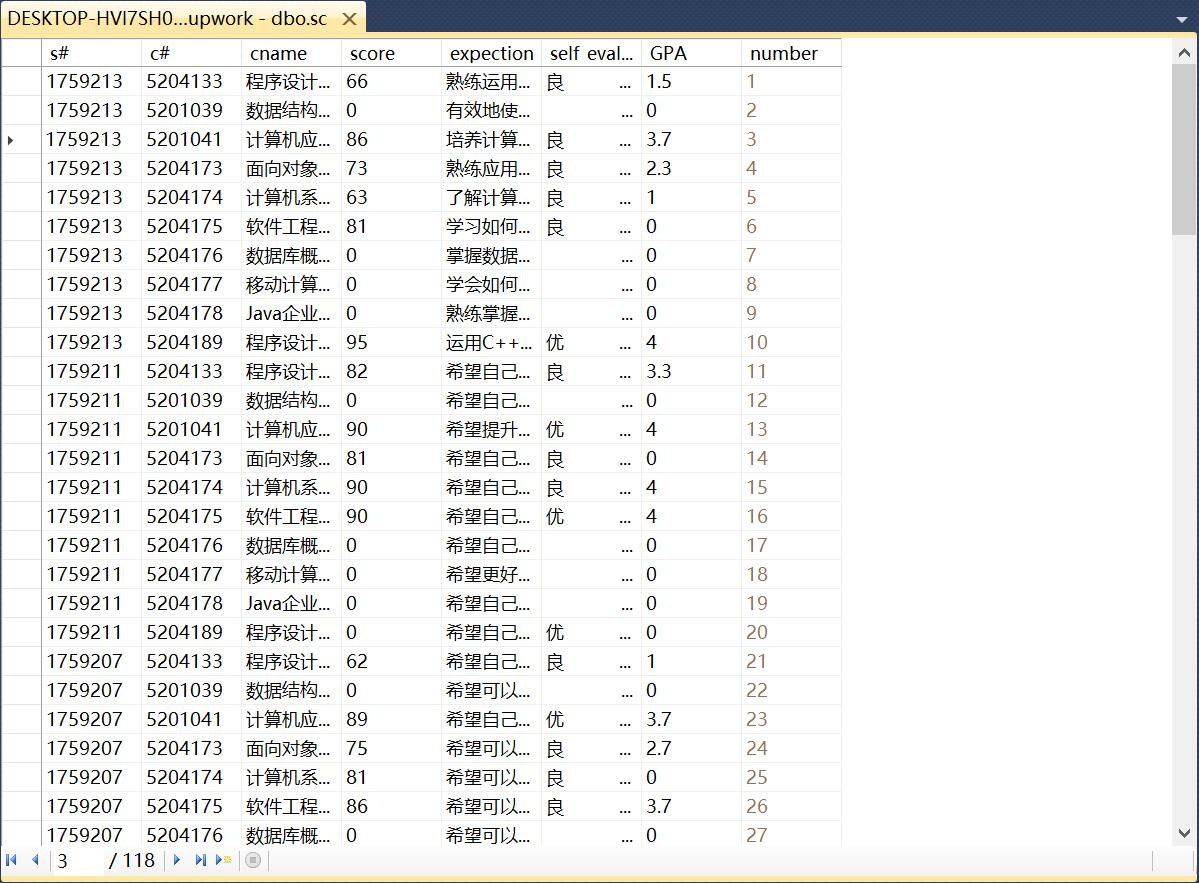## 【SQL Server】利用游标将学生表中的成绩转化为绩点```alter table sc
alter table sc
alter table sc add primary key(number)
declare score_visit cursor    --声明一个游标
for select score from sc
open score_visit              --打开游标
declare @GPA float
select @GPA=score from sc
fetch next from score_visit into @GPA
while @@fetch_status=0        --循环读取
begin
if @GPA>=90
update sc set GPA=4.0 where current of score_visit;
if @GPA >=85 and @GPA <90
update sc set GPA =3.7 where current of score_visit;
if @GPA >=82 and @GPA <85
update sc set GPA =3.3 where current of score_visit;
if @GPA >=78 and @GPA <81
update sc set GPA =3.0 where current of score_visit;
if @GPA >=75 and @GPA <78
update sc set GPA =2.7 where current of score_visit;
if @GPA >=72 and @GPA <75
update sc set GPA =2.3 where current of score_visit;
if @GPA >=68 and @GPA <72
update sc set GPA =2.0 where current of score_visit;
if @GPA >=64 and @GPA <68
update sc set GPA =1.5 where current of score_visit;
if @GPA >=60 and @GPA <64
update sc set GPA =1.0 where current of score_visit;
if @GPA <60
update sc set GPA =0 where current of score_visit;
fetch next from score_visit into @GPA
end
close score_visit              --关闭游标
deallocate score_visit         --删除游标```

游标：是一种能从包括多条数据记录的结果集中每次提取一条记录的机制。游标可以被看作是一个查询结果集（可以是零条、一条或由相关的选择 语句检索出的多条记录）和结果集中指向特定记录的游标位置组成的一个临时文件，提供了在查询结果集中向前或向后浏览数据处理结果集中数据的能力。

在表中加入一个GPA列并合理化声明一个游标后，将原先表中的成绩数据读取到一个新的变量中，利用绩点转换规则，将成绩转化为相应绩点，并生成到GPA列，操作完成后，关闭并删除游标。1.读取到的数据并不能进行实际操作

2.游标使用后未关闭/删除，导致程序下次运行时出现错误

3.成绩比较过程中的变量处理不恰当

1.将读取到的数据暂时放入到新声明的一个变量中

2.将使用后的游标关闭/删除

3.对变量进行规范处理，统一格式

1.将 if 所在的条件判断语句块进行简化

2.直接将读取数据进行转化，不必暂时放到新声明的变量内

3.在加入GPA列后对表中数据进行分段化处理，不至于显得杂乱